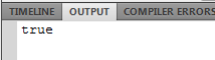LearnFast.Ninja

# As2 convert string to int – flash var string to number

How to make int from flash as2 string. Create some string variable. Convert this variable to int using the casting method. In the working example code we creating two variable with string numbers, then we convert then to int using cast int. After this process we can use the if statement to indicate which one of them is the biggest. Another option is to use the parseInt method.

Step 1: Create some string variable.
var a = "16";

Step 2: Convert this variable to int using the casting method.
a = int(a);

Another option: You can use parseInt.
a = parseInt(a);

Working code example:

var a = "16";

var b = "4";

a = int(a);

b = int(b);

trace(a > b);

Result:Tags

as2